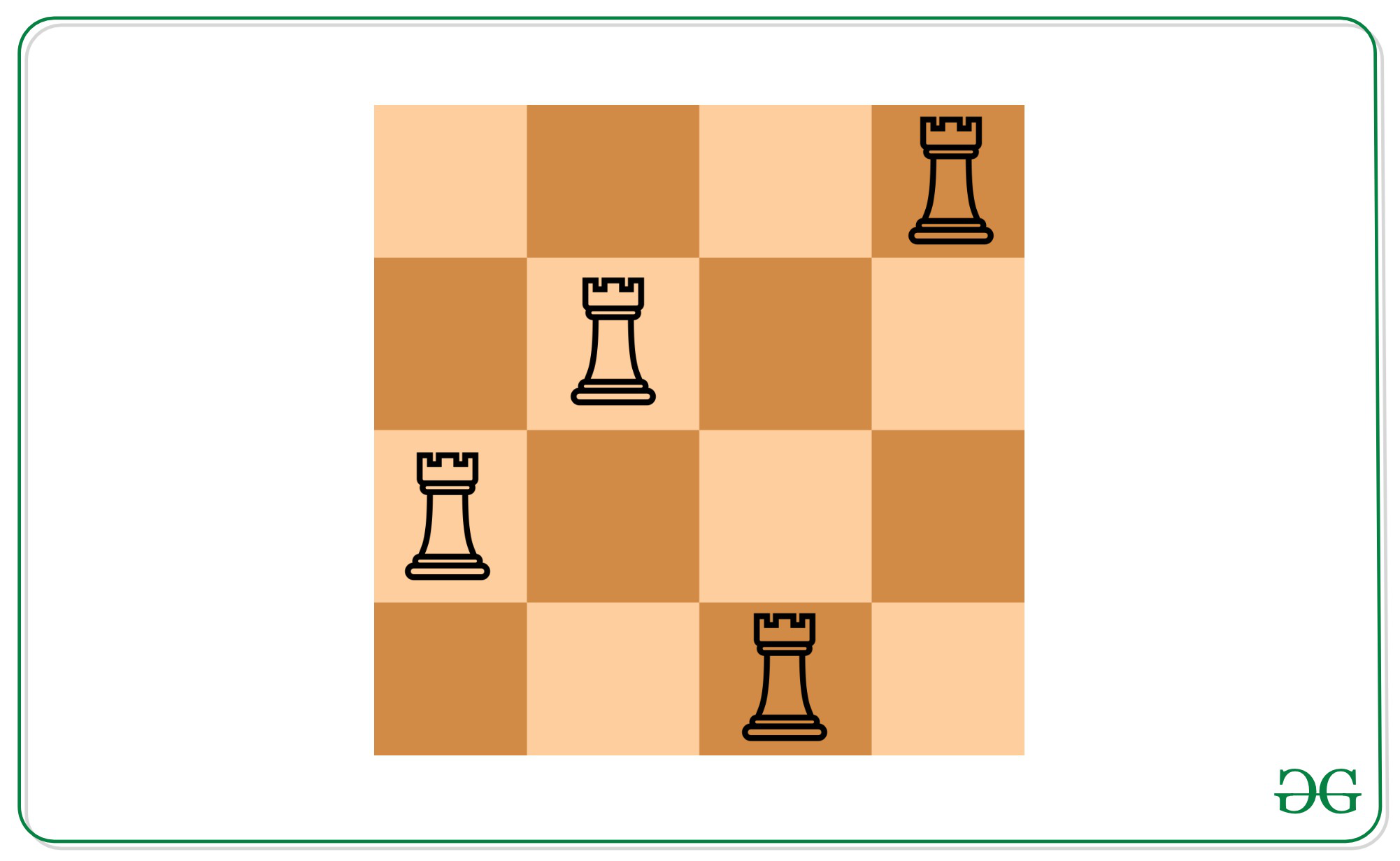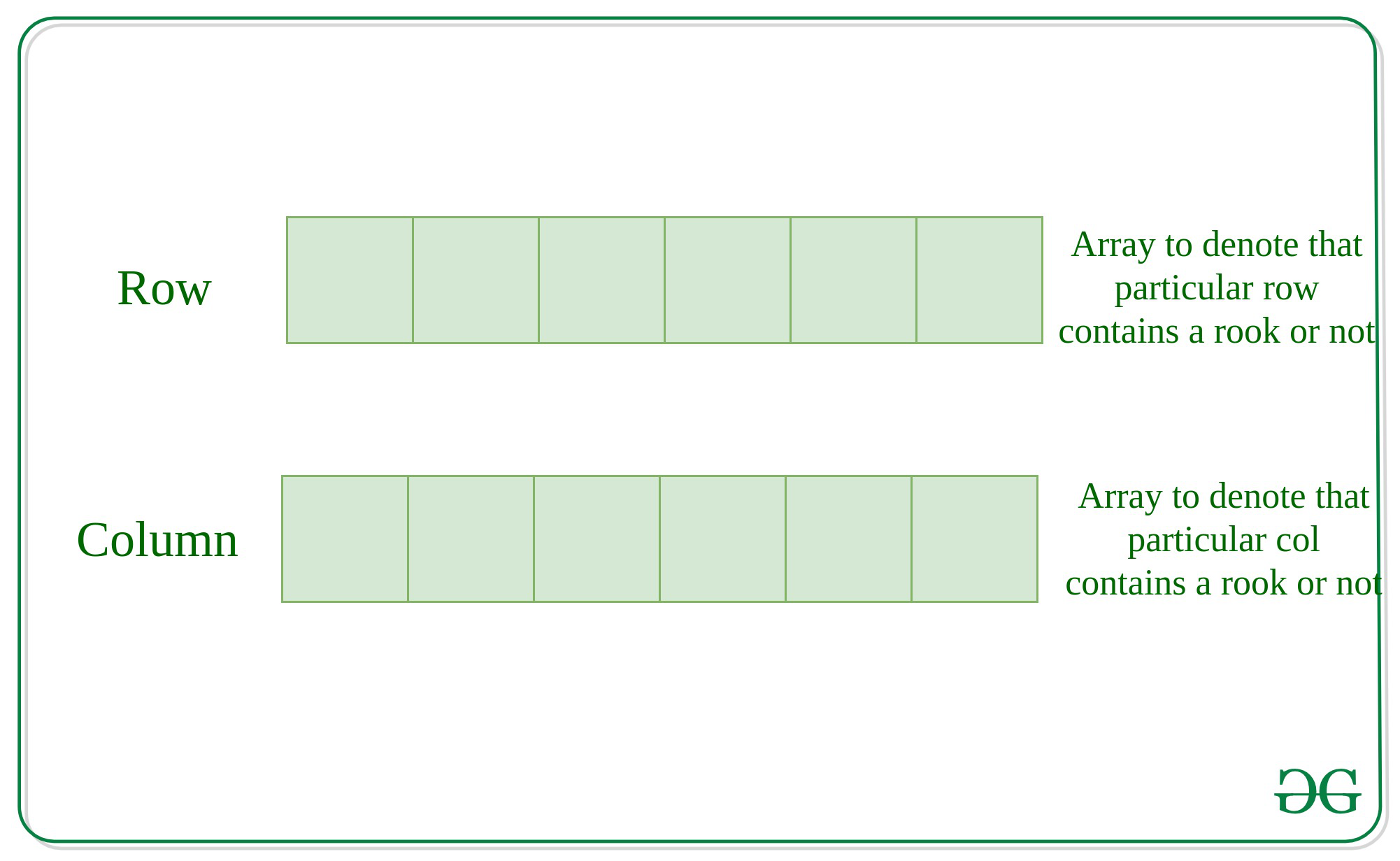# Find position of non-attacking Rooks in lexicographic order that can be placed on N*N chessboard

Given an integer N and an array arr[] of positions which denotes the positions of already placed non-attacking rooks, the task is to find the positions of non-attacking rooks in lexicographic order that can be placed on N*N chessboard.
Movement of Rooks: Any rook can move horizontally or vertically by any number of unoccupied squares.

Examples:

Inupt: N = 4, arr[] = {(1, 4), (2, 2)}
Output: 2
3 1
4 3
Explanation:
There can be two more rooks can be placed on the 4*4 ChessboardInput: N = 5, arr[] = {}
Output: 5
1 1
2 2
3 3
4 4
5 5

Naive Approach: Initialize a 2D Matrix mat[][] of size N*N with 0 at every cell and mark as rooks for initially positions of Rooks by 1.  Then traverse through matrix mat[][], checking whether ith row and jth column contains any rook,  Keeping a count of rooks placed. If any row contains and column both doesn’t contains any placed rook, then place a rook there and add this cell to your result String.
Finally, print count of rooks placed and positions of the rooks placement

Time Complexity: O(N3
Space Complexity: O(N2

Efficient Approach: The idea is to create two arrays of N size each, to store whether ith row or ith column contains any rook or not and it will now become efficient to search whether this row and the corresponding column already contains Rook or not.

Time Complexity: O(N2
Space Complexity: O(N)Most Efficient Approach:  The key observation in the problem is that the maximum rook that can be placed is N-K.  That is, Two rooks attack each other if they’re on the same row or on the same column. Since no two of the given rooks attack each other, all of the rows given in the input are unique. Similarly, all of the columns given in the input are unique. So, we’re left with N-K unused rows and N-K unused columns to put the new rooks on.
In other words, if we try to put more than N-K rooks by Pigeonhole Principle, if there are N+1 pigeons and N places to fill, then at least one place contains more than 1 pigeon.
And to find the lexicographically minimum answer. This answer can be achieved by pairing the smallest unused row with the smallest unused column, the second smallest unused row with the second smallest unused column and so on.
Time Complexity: O(N)

Below is the implementation of the above approach:

## C++

 `// C++ implementation to find ` `// count of placing non-attacking ` `// rooks on the N x N chessboard ` `#include ` `using` `namespace` `std; ` ` `  `// Function to find the count of ` `// placing non-attacking rooks ` `// on the N x N chessboard ` `void` `findCountRooks(``int` `row[], ``int` `col[],  ` `                    ``int` `n, ``int` `k) ` `{ ` `     `  `    ``// Count of the Non-attacking rooks ` `    ``int` `res = n - k; ` `    ``cout << res << ``"\n"``; ` `     `  `    ``int` `ri = 0, ci = 0; ` `     `  `    ``while` `(res-- > 0) ` `    ``{ ` `         `  `        ``// Printing lexographically ` `        ``// smallest configuration ` `        ``while` `(ri < k && row[ri] == 1) ` `        ``{ ` `            ``ri++; ` `        ``} ` `        ``while` `(ci < k && col[ci] == 1) ` `        ``{ ` `            ``ci++; ` `        ``} ` `        ``cout << (ri + 1) << ``" "`  `             ``<< (ci + 1) << ``"\n"``; ` `          `  `        ``ri++; ` `        ``ci++; ` `    ``} ` `} ` ` `  `// Driver Code ` `int` `main() ` `{ ` `    ``int` `n = 4; ` `    ``int` `k = 2; ` `    ``int` `row[] = { 1, 2 }; ` `    ``int` `col[] = { 4, 2 }; ` ` `  `    ``// Function call ` `    ``findCountRooks(row, col, n, k); ` `    ``return` `0; ` `} ` ` `  `// This code is contributed by jana_sayantan `

## Java

 `// Java implementation to find ` `// count of placing non-attacking ` `// rooks on the N x N chessboard ` ` `  `import` `java.util.Scanner; ` ` `  `public` `class` `P2Placerooks { ` `    ``// Function to find the count of ` `    ``// placing non-attacking rooks ` `    ``// on the N x N chessboard ` `    ``static` `void` `findCountRooks( ` `        ``int` `row[], ``int` `col[], ``int` `n, ``int` `k) ` `    ``{ ` ` `  `        ``// Count of the Non-attacking rooks ` `        ``int` `res = n - k; ` `        ``System.out.println(res + ``" "``); ` `        ``int` `ri = ``0``, ci = ``0``; ` `        ``while` `(res-- > ``0``) { ` ` `  `            ``// Printing lexographically ` `            ``// smallest configuration ` `            ``while` `(ri < k && row[ri] == ``1``) { ` `                ``ri++; ` `            ``} ` `            ``while` `(ci < k && col[ci] == ``1``) { ` `                ``ci++; ` `            ``} ` `            ``System.out.println((ri + ``1``) ` `                               ``+ ``" "` `+ (ci + ``1``) ` `                               ``+ ``" "``); ` `            ``ri++; ` `            ``ci++; ` `        ``} ` `    ``} ` ` `  `    ``// Driver Code ` `    ``public` `static` `void` `main(String[] args) ` `    ``{ ` `        ``int` `n = ``4``; ` `        ``int` `k = ``2``; ` `        ``int` `row[] = { ``1``, ``2` `}; ` `        ``int` `col[] = { ``4``, ``2` `}; ` ` `  `        ``// Function Call ` `        ``findCountRooks(row, col, n, k); ` `    ``} ` `} `

## Python3

 `# Python3 implementation to find ` `# count of placing non-attacking ` `# rooks on the N x N chessboard ` ` `  `# Function to find the count of ` `# placing non-attacking rooks ` `# on the N x N chessboard ` `def` `findCountRooks(row, col, n, k): ` `     `  `    ``# Count of the Non-attacking rooks ` `    ``res ``=` `n ``-` `k ` `    ``print``(res) ` `     `  `    ``ri ``=` `0` `    ``ci ``=` `0` `     `  `    ``while` `(res > ``0``): ` `         `  `        ``# Printing lexographically ` `        ``# smallest configuration ` `        ``while` `(ri < k ``and` `row[ri] ``=``=` `1``): ` `            ``ri ``+``=` `1` `         `  `        ``while` `(ci < k ``and` `col[ci] ``=``=` `1``): ` `            ``ci ``+``=` `1` `         `  `        ``print``((ri ``+` `1``), "", (ci ``+` `1``)) ` `         `  `        ``ri ``+``=` `1` `        ``ci ``+``=` `1` `        ``res ``-``=` `1` `     `  `# Driver Code ` `n ``=` `4` `k ``=` `2` ` `  `row ``=` `[ ``1``, ``2` `] ` `col ``=` `[ ``4``, ``2` `] ` ` `  `# Function call ` `findCountRooks(row, col, n, k) ` ` `  `# This code is contributed by sanjoy_62 `

## C#

 `// C# implementation to find ` `// count of placing non-attacking ` `// rooks on the N x N chessboard ` `using` `System; ` ` `  `class` `P2Placerooks{ ` `     `  `// Function to find the count of ` `// placing non-attacking rooks ` `// on the N x N chessboard ` `static` `void` `findCountRooks(``int` `[]row, ` `                           ``int` `[]col, ` `                           ``int` `n, ``int` `k) ` `{ ` `     `  `    ``// Count of the Non-attacking rooks ` `    ``int` `res = n - k; ` `    ``Console.WriteLine(res + ``" "``); ` `     `  `    ``int` `ri = 0, ci = 0; ` `    ``while` `(res-- > 0) ` `    ``{ ` `         `  `        ``// Printing lexographically ` `        ``// smallest configuration ` `        ``while` `(ri < k && row[ri] == 1) ` `        ``{ ` `            ``ri++; ` `        ``} ` `        ``while` `(ci < k && col[ci] == 1) ` `        ``{ ` `            ``ci++; ` `        ``} ` `        ``Console.WriteLine((ri + 1) + ``" "` `+ ` `                          ``(ci + 1) + ``" "``); ` `        ``ri++; ` `        ``ci++; ` `    ``} ` `} ` ` `  `// Driver Code ` `public` `static` `void` `Main(String[] args) ` `{ ` `    ``int` `n = 4; ` `    ``int` `k = 2; ` `    ``int` `[]row = { 1, 2 }; ` `    ``int` `[]col = { 4, 2 }; ` ` `  `    ``// Function call ` `    ``findCountRooks(row, col, n, k); ` `} ` `} ` ` `  `// This code is contributed by Rajput-Ji `

Output:

```2
2 1
3 2
```

Performance Analysis:

• Time Complexity: O(N)
• Auxiliary Space: O(1)

Attention reader! Don’t stop learning now. Get hold of all the important DSA concepts with the DSA Self Paced Course at a student-friendly price and become industry ready.

My Personal Notes arrow_drop_upCheck out this Author's contributed articles.

If you like GeeksforGeeks and would like to contribute, you can also write an article using contribute.geeksforgeeks.org or mail your article to contribute@geeksforgeeks.org. See your article appearing on the GeeksforGeeks main page and help other Geeks.

Please Improve this article if you find anything incorrect by clicking on the "Improve Article" button below.